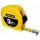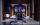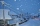# Fraction calculator

The calculator performs basic and advanced operations with fractions, expressions with fractions combined with integers, decimals, and mixed numbers. It also shows detailed step-by-step information about the fraction calculation procedure. Solve problems with two, three, or more fractions and numbers in one expression.

## Result:

### 1/5 + 3/4 + 1/2 = 29/20 = 1 9/20 = 1.45

Spelled result in words is twenty-nine twentieths (or one and nine twentieths).

### How do you solve fractions step by step?

1. Add: 1/5 + 3/4 = 1 · 4/5 · 4 + 3 · 5/4 · 5 = 4/20 + 15/20 = 4 + 15/20 = 19/20
For adding, subtracting, and comparing fractions, it is suitable to adjust both fractions to a common (equal, identical) denominator. The common denominator you can calculate as the least common multiple of both denominators - LCM(5, 4) = 20. In practice, it is enough to find the common denominator (not necessarily the lowest) by multiplying the denominators: 5 × 4 = 20. In the following intermediate step, the fraction result cannot be further simplified by canceling.
In other words - one fifth plus three quarters = nineteen twentieths.
2. Add: the result of step No. 1 + 1/2 = 19/20 + 1/2 = 19/20 + 1 · 10/2 · 10 = 19/20 + 10/20 = 19 + 10/20 = 29/20
For adding, subtracting, and comparing fractions, it is suitable to adjust both fractions to a common (equal, identical) denominator. The common denominator you can calculate as the least common multiple of both denominators - LCM(20, 2) = 20. In practice, it is enough to find the common denominator (not necessarily the lowest) by multiplying the denominators: 20 × 2 = 40. In the following intermediate step, the fraction result cannot be further simplified by canceling.
In other words - nineteen twentieths plus one half = twenty-nine twentieths.

#### Rules for expressions with fractions:

Fractions - use the slash “/” between the numerator and denominator, i.e., for five-hundredths, enter 5/100. If you are using mixed numbers, be sure to leave a single space between the whole and fraction part.
The slash separates the numerator (number above a fraction line) and denominator (number below).

Mixed numerals (mixed fractions or mixed numbers) write as non-zero integer separated by one space and fraction i.e., 1 2/3 (having the same sign). An example of a negative mixed fraction: -5 1/2.
Because slash is both signs for fraction line and division, we recommended use colon (:) as the operator of division fractions i.e., 1/2 : 3.

Decimals (decimal numbers) enter with a decimal point . and they are automatically converted to fractions - i.e. 1.45.

The colon : and slash / is the symbol of division. Can be used to divide mixed numbers 1 2/3 : 4 3/8 or can be used for write complex fractions i.e. 1/2 : 1/3.
An asterisk * or × is the symbol for multiplication.
Plus + is addition, minus sign - is subtraction and ()[] is mathematical parentheses.
The exponentiation/power symbol is ^ - for example: (7/8-4/5)^2 = (7/8-4/5)2

#### Examples:

subtracting fractions: 2/3 - 1/2
multiplying fractions: 7/8 * 3/9
dividing Fractions: 1/2 : 3/4
exponentiation of fraction: 3/5^3
fractional exponents: 16 ^ 1/2
adding fractions and mixed numbers: 8/5 + 6 2/7
dividing integer and fraction: 5 ÷ 1/2
complex fractions: 5/8 : 2 2/3
decimal to fraction: 0.625
Fraction to Decimal: 1/4
Fraction to Percent: 1/8 %
comparing fractions: 1/4 2/3
multiplying a fraction by a whole number: 6 * 3/4
square root of a fraction: sqrt(1/16)
reducing or simplifying the fraction (simplification) - dividing the numerator and denominator of a fraction by the same non-zero number - equivalent fraction: 4/22
expression with brackets: 1/3 * (1/2 - 3 3/8)
compound fraction: 3/4 of 5/7
fractions multiple: 2/3 of 3/5
divide to find the quotient: 3/5 ÷ 2/3

The calculator follows well-known rules for order of operations. The most common mnemonics for remembering this order of operations are:
PEMDAS - Parentheses, Exponents, Multiplication, Division, Addition, Subtraction.
BEDMAS - Brackets, Exponents, Division, Multiplication, Addition, Subtraction
BODMAS - Brackets, Of or Order, Division, Multiplication, Addition, Subtraction.
GEMDAS - Grouping Symbols - brackets (){}, Exponents, Multiplication, Division, Addition, Subtraction.
Be careful, always do multiplication and division before addition and subtraction. Some operators (+ and -) and (* and /) has the same priority and then must evaluate from left to right.

## Fractions in word problems:

• Conversion of unitsComplete the following length data
• Roses and tulipsAt the florist are 50 tulips and 5 times fewer roses. How many flowers are in the flower shop?
• CupcakesIn a bowl was some cupcakes. Janka ate one third and Danka ate one quarter of cupcakes. a) How many of cookies ate together? b) How many cookies remain in a bowl? Write the results as a decimal number and in notepad also as a fraction.
• Length subtractingExpress in mm: 5 3/10 cm - 2/5 mm
• EvaluateThe division of numbers \$a and \$b increase by-product of the numbers \$c and \$d
• Stones in aquariumIn an aquarium with a length of 2 m, 1.5 m wide, and 2.5 m deep, the water is up to three-quarters of the depth. Can we place 2m cubic meters of stones in the aquarium without spilling water? (0 = no, 1 = yes)
• FractionFraction ? write as fraction a/b, a, b is integers numerator/denominator.
• Master and apprenticeMaster painted the roof in 3 hours and apprentice for 4 hours. How many of roof they painted in hour and how many in three quarters of an hour?
• Infinite sum of areasAbove the height of the equilateral triangle ABC is constructed an equilateral triangle A1, B1, C1, of the height of the equilateral triangle built A2, B2, C2, and so on. The procedure is repeated continuously. What is the total sum of the areas of all tr
• Sum three fractionsWork out the sum of 1/4, 1/5 and 3/10.Max is working out 2/3+7/9. He says the answer is 9/12. What mistake have Max made?7 is added to the sum of 4/5 and 6/7Samuel has 1/3 of a bag of rice and Isabella has a 1/2 bag of rice. What fraction of are bag of rice do they have altogether?